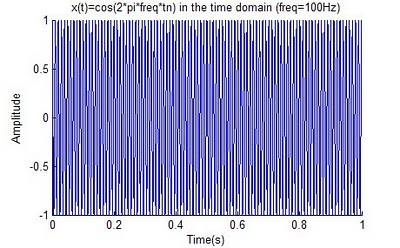## Sunday, February 14, 2010

### DSP Tutorial #6: Looping in MatlabLooping is helpful if we need to repeat certain specific commands. In this example we will use the for loop to create a sinusoid of various frequencies as a function of the loop variable. The following code creates six (6) plots, each with a different 'frequency' as determined by the conditional if statement based on our loop variable i.

close all;
clear all;
for i=1:6;
if i==1,freq=10;,end;
if i==2,freq=25;,end;
if i==3,freq=50;,end;
if i==4,freq=75;,end;
if i==5,freq=100;,end;
if i==6,freq=125;,end;
fs = 8000;
Ts=1/fs; % sample period
n=0:1*fs; %sample index (fs=samples per second), 1 second
tn=n*Ts;
x=cos(2*pi*freq*tn);
% Time domain plot of x(t)=cos(2*pi*5*tn)
figure('Color',[1 1 1]);
h=plot(tn,x);box off;
xlabel('Time(s)');
ylabel('Amplitude');
title(['x(t)=cos(2*pi*freq*tn) in the time domain (freq=',num2str(freq),'Hz)']);
end;